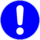# MC-Basic:element.SMOOTHFACTOR

This property is used to control the amount of smoothing applied to the s-curve profile. The smooth factor may be set to a value in the range 1 to 100, to correspond approximately to an acceleration time ranging from V/A to 3*V/A (where V is the cruise velocity and A is the acceleration).

Setting the factor to 0 engages the trapezoidal velocity with no smoothing.

Setting the factor to -1 returns jerk control, in which the exact value of JERK is set. When SMOOTHFACTOR is set to -1, the JERK property is used to control the amount of smoothing.

This property is used inside a motion command to override the permanent value.IMPORTANT If MODAL value of the PrfType equals -1 (default from 4.7.3!) the SmoothFactor is the only one who determines the profile type. Values of the SmoothFactor between [1,100] and the value of -1 define the SINE-ACCELERATION profile and value of zero the VELOCITY-TRAPEZE profile. If MODAL value of PrfType differs from -1, SmoothFactor has no influence on the type of the profile. Still the SmoothFactor defines the JERK value (as before).

<element>.Smooth

## Syntax

<element>.SmoothFactor = <expression>

?<element>.SmoothFactor

All versions

Double

-1, 0, 1 to 100

1

## Limitations

To set the value within a task, the element must be attached to that task (using the ATTACH command).

## Examples

Axis
A1.Smoothfactor = 30

Move A1 100 SmoothFactor = 20

Group
G1.Smoothfactor = 30
Move G1 {100, 200} SmoothFactor = 20